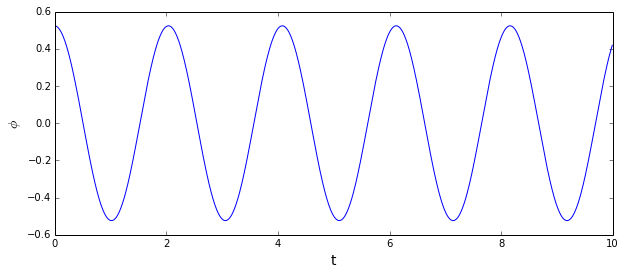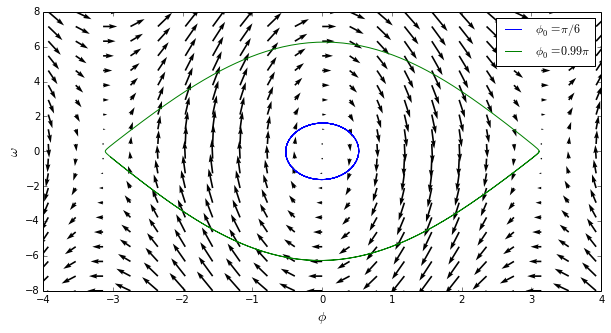This notebook is inspired by watching the videos for Underactuated Robotics MIT lectures and edx course. The videos of the example robots in the course were very cool (look for cart pole swingup and acrobot videos on youtube). Unfortunately I had already committed myself to a couple of different MOOCs while Underactuated Robotics was running, so couldn't dedicate enough time to keep up, but I want to dig into the material at a more leisurely pace, as it's still available online.

I wanted to investigate some of the ideas in the course, and brush up on the areas I'm weak on. Time permitting, I'm planning to code up some of the concepts in the course.

I discovered iPython notebooks via the Practical Numerical Methods with Python MOOC, and it's a great idea to create a digital copy of my notes and the algorithms via code.

# Undamped Pendulum Dynamics¶

The dynamics of an simple undamped is given in the following equation

The angular acceleration $\frac{d^2{\phi}}{dt^2}={\alpha}(t)=-\frac{g}{l} {sin{\phi(t)}}$

The angular velocity is ${\omega}(t)$. Euler integration gives:

$$\begin{eqnarray} {\omega}(t + {\Delta}t) = {\omega}(t) + {\Delta}t.{\alpha}(t) \\ {\phi}(t + {\Delta}t) = {\phi}(t) + {\Delta}t.{\omega}(t) \end{eqnarray}$$

# Coding¶

This is simple to code up. First, import the required libraries.

In :
import numpy
import math
from matplotlib import pyplot
%matplotlib inline


The method simulate_pendulum initialises the result arrays, and the for loop performs an Euler integration over the time period.

In :
# constants
g = 9.81
l = 1.0

def simulate_pendulum(phi_init, omega_init, numSteps, deltaT):
t = numpy.zeros(numSteps)

phi = numpy.zeros(numSteps)
phi = phi_init

omega = numpy.zeros(numSteps)
omega = omega_init

for n in range(1,numSteps):
t[n] = deltaT * n # store the time for plotting.

alpha_t = -(g/l) * math.sin(phi[n-1])
omega_t = omega[n-1] + (delta_t * alpha_t)
phi_t = phi[n-1] + (delta_t * omega_t)
omega[n] = omega_t
phi[n] = phi_t

return (t, phi, omega)


The method can be ran for a set of initial conditions, and the results stored in a tuple of time against angle and angular velocity.

In :
# initial conditions
phi_0 = math.pi / 6.  # initial angle
omega_0  = 0.         # pendulum initially at rest

N = 1000              # time steps to run the simulation for
delta_t = 0.01        # simulation step size

(t, phi, omega) = simulate_pendulum(phi_0, omega_0, N, delta_t)


Plotting the results shows the expected periodic motion.

In :
pyplot.figure(figsize=(10,4))
pyplot.xlabel('t', fontsize=14)
pyplot.ylabel(r'$\phi$', fontsize=14)
pyplot.hold(True)
pyplot.plot(t, phi)

Out:
[<matplotlib.lines.Line2D at 0x7f08fce8fb10>]# Phase Portrait¶

A phase portrait of the undamped pendulum can be generated.

The state vector of the pendulum is:

$$\mathbf{x} = \begin{bmatrix} {\phi} \\ {\dot{\phi}} \\ \end{bmatrix}$$

and the phase portrait is a plot of the trajectories at each point in the state space, i.e.

$$\dot{\mathbf{x}} = \begin{bmatrix} \dot{{\phi}} \\ \ddot{{\phi}} \\ \end{bmatrix} = \begin{bmatrix} {\omega} \\ -\frac{g}{l} {sin{\phi(t)}} \\ \end{bmatrix}$$

The phase portrait can be plotted with the following code:

In :
# this gives the trajectories - the derivative of the state-space matrix
def dX_dt(X):
#phi = X
#omega = X = phi_dot
#omega_dot = -(g/l)*math.sin(phi)
#return phi_dot, omega_dot
return numpy.array([ X, -(g/l)*numpy.sin(X) ]);

x = numpy.linspace(-math.pi*1.25, math.pi*1.25, 21)
y = numpy.linspace(-8, 8, 20)

(X,Y) = numpy.meshgrid(x, y)
(DX, DY) = dX_dt([X, Y])

pyplot.figure(figsize=(10,5))
pyplot.xlabel(r'$\phi$', fontsize=14)
pyplot.ylabel(r'$\omega$', fontsize=14)
pyplot.quiver(X, Y, DX, DY)

# simulate a second trajectory with a larger initial angle
(t2, phi_large, omega_large) = simulate_pendulum(math.pi * 0.99, 0, N, delta_t)

coords = zip(omega, phi)
pyplot.hold(True)
pyplot.plot(phi, omega, color='b', label='$\phi_0=\pi/6$')
pyplot.plot(phi_large, omega_large, color='g', label='$\phi_0=0.99\pi$')
pyplot.legend()

Out:
<matplotlib.legend.Legend at 0x7f08fc762fd0>This shows the stable fixed point when ${\phi}$ is zero and $\dot{\phi}$ is zero, and the unstable fixed points where $\dot{\phi}$ is zero and ${\phi}$ is at $\pm{\pi}$ radians (where the pendulum is balanced in the top position).

# Animation¶

The pendulum can be plotted over time using the JSAnimation library, using the trajectory that has already been calculated.

In :
from JSAnimation import IPython_display
from matplotlib import animation

fig = pyplot.figure(figsize=(8,5))
ax = pyplot.axes(xlim=(0,2), ylim=(-0.2,2))
line, = pyplot.plot([],[])
time_text = pyplot.text(0.02, 1.90, '')

def animate(i):
phi_step = phi[i]
line.set_data([1,1-math.sin(phi_step)],[1,1-math.cos(phi_step)])
time_text.set_text('time = %.1f' % t[i])

animation.FuncAnimation(fig, animate,
frames=200, interval=20)

Out:

Once Loop Reflect